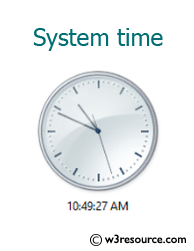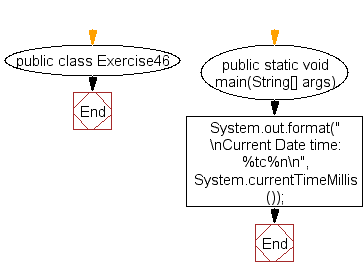﻿ Java - Display the system time

# Java: Display the system time

## Java Basic: Exercise-46 with Solution

Write a Java program to display system time.

Pictorial Presentation:Sample Solution:

Java Code:

``````public class Exercise46 {

public static void main(String[] args){
System.out.format("\nCurrent Date time: %tc%n\n", System.currentTimeMillis());
}
}
```
```

Sample Output:

```Current Date time: Fri Jun 16 14:17:40 IST 2017
```

Flowchart:Java Code Editor:

What is the difficulty level of this exercise?

Test your Programming skills with w3resource's quiz.

﻿

## Java: Tips of the Day

Oddness Check

Is it possible to use this code to accurately determine the odd number?

```public boolean oddOrNot(int num) {
return num % 2 == 1;
}
```

I hope you noticed a trick. If we decide to check a negative odd number in such a way (-5, for example), the remainder of the division will not be equal to one (what does it mean?) Therefore, use a more accurate method:

```public boolean oddOrNot(int num) {
return (num & 1) != 0;
}
```

It not only solves the problem of negative numbers, but also works more productively than its predecessor. Arithmetic and logical operations are performed much faster than multiplication and division.

Ref: https://bit.ly/3giyonA

We are closing our Disqus commenting system for some maintenanace issues. You may write to us at reach[at]yahoo[dot]com or visit us at Facebook